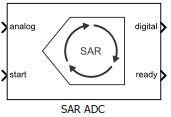N-bit successive approximation register (SAR) based ADC

• Library:
• Mixed-Signal Blockset / ADC / Architectures

•## Description

Successive Approximation Register (SAR) based ADC consists of a sample and hold circuit (SHA), a comparator, an internal digital to analog converter (DAC), and a successive approximation register.When the ADC receives the start command, SHA is placed in hold mode. The most significant bit (MSB) of the SAR is set to logic `1`, and all other bits are set to logic `0`.

The output of the SAR is fed back to a DAC, whose output is compared with the incoming input signal. If the DAC output is greater than the analog input, MSB is reset, otherwise it is left set. The next MSB is now set to 1, and the process is repeated until every bit the SAR is compared. The final value of the SAR at the end of this process corresponds to the analog input value. The end of the conversion process is indicated by the ready signal.

## Ports

### Input

expand all

Data Types: `double`

External conversion start clock, specified as a scalar. start determines when the analog to digital conversion process starts.

Data Types: `double`

### Output

expand all

Converted digital output signal, returned as a scalar.

Data Types: `fixed point` | `single` | `double` | `int8` | `int16` | `int32` | `uint8` | `uint16` | `uint32` | `Boolean`

Determines whether the analog to digital conversion is complete, returned as a scalar.

Data Types: `double`

## Parameters

expand all

### Configuration

Number of physical output bits, specified as a unitless positive real integer in the range `[1, 26]`. Number of bits determines the resolution of the ADC.

#### Programmatic Use

• Use `get_param(gcb,'NBits')` to view the current Number of bits.

• Use `set_param(gcb,'NBits',value)` to set Number of bits to a specific value.

Data Types: `double`

ADC dynamic range, specified as a 2-element row vector in volts.

#### Programmatic Use

• Use `get_param(gcb,'InputRange')` to view the current Input range (V).

• Use `set_param(gcb,'InputRange',value)` to set Input range (V) to a specific value.

Data Types: `double`

Select to connect to an external start conversion clock. By default, this option is selected. If you deselect this option, a Sampling Clock Source block inside the Flash ADC is used to generate the start conversion clock.

Frequency of internal start conversion clock, specified as a positive real scalar in Hz. Conversion start frequency determines the rate of the ADC.

#### Dependencies

This parameter is only available when Use external start clock is not selected.

#### Programmatic Use

• Use `get_param(gcb,'StartFreq')` to view the current value of Conversion start frequency (Hz).

• Use `set_param(gcb,'StartFreq',value)` to set Conversion start frequency (Hz) to a specific value.

Data Types: `double`

RMS aperture jitter added as an impairment to the start conversion clock, specified as a real nonnegative scalar in s. Set RMS aperture jitter value to zero if you want a clean clock signal.

#### Dependencies

This parameter is only available when Use external start clock is not selected.

#### Programmatic Use

• Use `get_param(gcb,'StartClkJitter')` to view the current value of RMS aperture jitter (s).

• Use `set_param(gcb,'StartClkJitter',value)` to set RMS aperture jitter (s) to a specific value.

Data Types: `double`

Frequency of the SAR clock, specified as a real scalar in Hz. SAR Frequency (Hz) must be high enough to allow the ADC to perform Nbits comparison, where Nbits is the Number of bits of the ADC. The block has one cycle overhead due to algebraic loop removal. So, the clock must run for one additional cycle before the output is ready. So, the SAR Frequency (Hz) (fSAR) is given by the equation ${f}_{\text{SAR}}\ge \left(Nbits+1\right){f}_{\text{start}}$, where fstart is the Conversion start frequency.

#### Programmatic Use

• Use `get_param(gcb,'SARFreq')` to view the current value of SAR Frequency (Hz).

• Use `set_param(gcb,'SARFreq',value)` to set SAR Frequency (Hz) to a specific value.

Inherit the output polarity and data type from the analog input signal to the ADC. When this option is selected, it forces the ADC to output a scalar double matched to the input's scale of the ADC.

Defines the ADC output data polarity.

If Output polarity is set to `Auto`, the minimum and maximum values of the output are determined by the polarity of the Input range.

If Output polarity is set to `Bipolar`, the outputs are between `-2Nbits-1` and `2Nbits-1-1`.

If Output polarity is set to `Unipolar`, the outputs are between `0` and `2Nbits-1`.

#### Dependencies

This parameter is only editable when Match input scale option is deselected.

#### Programmatic Use

• Use `get_param(gcb,'OutputPolarity')` to view the current Output polarity.

• Use `set_param(gcb,'OutputPolarity',value)` to set Output polarity to a specific value.

Unsigned integers and fixed-point types (`fixdt(0,Nbits)`) are not available when the Output polarity is set to `Bipolar` or `Auto`.

Signed integers and fixed-point types (`fixdt(1,Nbits)`) are not available when the Output polarity is set to `Unipolar`.

#### Dependencies

This parameter is only editable when Match input scale option is deselected.

#### Programmatic Use

• Use `get_param(gcb,'OutDataType')` to view the current Output data type.

• Use `set_param(gcb,'OutDataType',value)` to set Output data type to a specific value.

### Impairments

Select to enable impairments such as offset error and gain error in ADC simulation. By default, this option is deselected.

Shifts quantization steps by specific value, specified as a scalar in least significant bit (LSB) or %.

#### Dependencies

This parameter is only available when Enable impairments is selected.

#### Programmatic Use

• Use `get_param(gcb,'OffsetError')` to view the current value of Offset error (LSB).

• Use `set_param(gcb,'OffsetError',value)` to set Offset error (LSB) to a specific value.

Data Types: `double`

Error on the slope of the straight line interpolating ADC transfer curve, specified as a real scalar in %FS, FS, or LSB.

#### Dependencies

This parameter is only available when Enable impairments is selected in the Impairments tab.

#### Programmatic Use

• Use `get_param(gcb,'GainError')` to view the current value of Gain error.

• Use `set_param(gcb,'GainError',value)` to set Gain error to a specific value.

Data Types: `double`